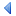UpTOC Index << 3 Using Euphoria < 4.6 Flow control statements Up: 4 Language Reference 4.8 Special Top-Level Statements > 5 Formal Syntax >>

## 4.7 Short-Circuit Evaluation

When the condition tested by if, elsif, until, or while contains and or or operators, short_circuit evaluation will be used. For example,

```if a < 0 and b > 0 then ...
```

If a < 0 is false, then Euphoria will not bother to test if b is greater than 0. It will know that the overall result is false regardless. Similarly,

```if a < 0 or b > 0 then ...
```

if a < 0 is true, then Euphoria will immediately decide that the result is true, without testing the value of b, since the result of this test would be irrelevant.

In general, whenever we have a condition of the form:

```A and B
```

where A and B can be any two expressions, Euphoria will take a short-cut when A is false and immediately make the overall result false, without even looking at expression B.

Similarly, with:

```A or B
```

when A is true, Euphoria will skip the evaluation of expression B, and declare the result to be true.

If the expression B contains a call to a function, and that function has possible side-effects, i.e. it might do more than just return a value, you will get a compile-time warning. Older versions (pre-2.1) of Euphoria did not use short_circuit evaluation, and it's possible that some old code will no longer work correctly, although a search of the Euphoria archives did not turn up any programs that depend on side-effects in this way, but other Euphoria code might do so.

The expression, B, could contain something that would normally cause a run-time error. If Euphoria skips the evaluation of B, the error will not be discovered. For instance:

```if x != 0 and 1/x > 10 then  -- divide by zero error avoided

while 1 or {1,2,3,4,5} do    -- illegal sequence result avoided
```

B could even contain uninitialized variables, out-of-bounds subscripts etc.

This may look like sloppy coding, but in fact it often allows you to write something in a simpler and more readable way. For instance:

```if length(x) > 1 and x = y then
```

Without short-circuiting, you would have a problem when x contains less than 2 items. With short-circuiting, the assignment to x will only be done when x has at least 2 items. Similarly:

```-- find 'a' or 'A' in s
i = 1
while i <= length(s) and s[i] != 'a' and s[i] != 'A' do
i += 1
end while
```

In this loop the variable i might eventually become greater than length(s). Without short-circuit evaluation, a subscript out-of-bounds error will occur when s[i] is evaluated on the final iteration. With short-circuiting, the loop will terminate immediately when i <= length(s) becomes false. Euphoria will not evaluate s[i] != 'a' and will not evaluate s[i] != 'A'. No subscript error will occur.

Short-circuit evaluation of and and or takes place inside decision making expressions. These are found in the if statement, while statement and the loop until statement. It is not used in other contexts. For example, the assignment statement:

```x = 1 or {1,2,3,4,5}  -- x should be set to {1,1,1,1,1}
```

If short-circuiting were used here, we would set x to 1, and not even look at {1,2,3,4,5}. This would be wrong. Short-circuiting can be used in if/elsif/until/while conditions because we only care if the result is true or false, and conditions are required to produce an atom as a result.UpTOC Index << 3 Using Euphoria < 4.6 Flow control statements Up: 4 Language Reference 4.8 Special Top-Level Statements > 5 Formal Syntax >>